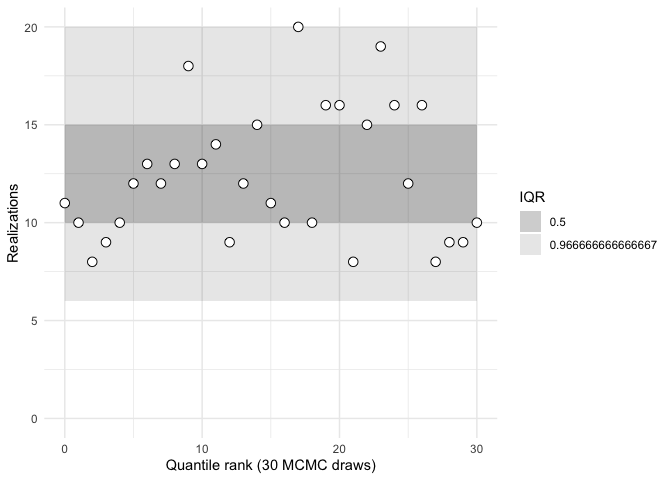This package provides tools to simplify the implementation of simulation based calibration using rank statistics (Talts, Betancourt, Simpson, Vehtari, and Gelman, arXiv:1804.06788). It implements a very similar validation procedure to that in rstan::sbc but using a different workflow. In rstan::sbc, the Stan model must be modified by the user to generate rank statistics during sampling. In this package, the Stan model is left unmodified, and the code needed to calculate the rank statistics is written in R. This provides a potentially faster development workflow (Stan recompiles are not needed). It also allows SBC to be used in cases where generating parameters from their prior distributions, or the modeled data from the likelihood function, would be too complicated if written in Stan.

## Installation

You can install the development version from GitHub with:

# install.packages("devtools")
devtools::install_github("jasonmtroos/sbcrs")

To build the package vignettes, install the package using:

devtools::install_github("jasonmtroos/sbcrs", build_vignettes = TRUE)

The package vignettes are a useful starting point for understanding what this package does:

• intro-to-sbc provides an overview to simulation-based calibration, and the features of the SBC package

• funnel shows how SBC identified sampling problems in Neal’s funnel

• comparison-to-rstan-sbc implements the example from rstan::sbc using this package, shows the rank statistics are the same, and provides a basis for understanding the different design philosophies behind the two approaches.

It is also useful to understand why this package does what it does. For that, see: Validating Bayesian Inference Algorithms with Simulation-Based Calibration, arXiv:1804.06788.

## A Simple Illustration of What This Thing Does

library(rstan)
my_model <- stan_model(model_code = "
data {
vector y;
vector x;
vector w;
}
parameters {
real alpha;
real beta;
real<lower = 0> sigma;
}
model {
alpha ~ std_normal();
beta ~ std_normal();
sigma ~ exponential(1);
y ~ normal(alpha + beta * x + beta^2 * w, sigma);
}
")

How can we be sure this Stan model is able to sample from the posterior distribution of alpha, beta, and sigma? Read the paper!

Here’s the code:

library(sbcrs)
doParallel::registerDoParallel(parallel::detectCores())
x <- sample.int(5, 100, replace = TRUE)
w <- rnorm(100)
my_sbc <- SBC$new( data = function(seed) { list(w = w, x = x) }, params = function(seed, data) { set.seed(seed + 1e6) alpha <- rnorm(1) beta <- rnorm(1) sigma <- rexp(1) list(alpha = alpha, beta = beta, sigma = sigma) }, modeled_data = function(seed, data, params) { set.seed(seed + 2e6) list(y = rnorm(100, params$alpha +
params$beta * data$x +
params$beta^2 * data$w, params$sigma)) }, sampling = function(seed, data, params, modeled_data, iters) { data_for_stan <- c(data, modeled_data) rstan::sampling(my_model, data = data_for_stan, seed = seed, chains = 1, iter = 2 * iters, warmup = iters, refresh = 200) } ) my_sbc$calibrate(N = 128, L = 30, keep_stan_fit = FALSE)
my_sbc\$plot()Looks good!

## Contributing

Please note that the ‘sbcrs’ project is released with a Contributor Code of Conduct. By contributing to this project, you agree to abide by its terms.